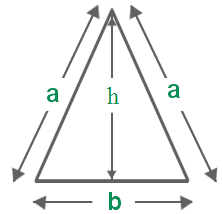# C Program Area Of Isosceles Triangle | C Programs

C Program to find the area of an isosceles triangle – In this specific article, we will detail in on the several methods used to calculate the area of an isosceles triangle in C programming.

Check out the complete blog to understand the ways. The compiler has been added to aid you with the solutions. Also, check out the sample programs and suitable examples to calculate the area of an isosceles triangle.

The ways used to calculate the area of an isosceles triangle in C programming are as follows:

• Using Standard Method
• Using Function
• Using Pointers
• Using Macros

As we all know, an isosceles triangle is a triangle in which a couple of its sides are equal. Because of this specific trait, the angles opposite to the equal sides are also equal in nature.

An isosceles triangle looks like this:As you can see in the image uploaded above, the isosceles triangle has a couple of sides with equal length a. b is the base and h is the height of the isosceles triangle.

The area of an isosceles triangle calculated with the help of this formula:

Area = 1/2 * Base * Height.

Thus, all the methods used to calculate the area of the isosceles triangle are as follows:

## Using Standard Method

1) Length of same-sided will be store into “a” variable

2) Side2 value will be store in to “b” variable

3) Now we are calculating the area using area=(b*sqrt(4*(a*a)-(b*b)))/4

output:

### Using Function

1)We are calling the function area(a,b) by passing a,b values

2)Now  float area(float a, float b) function  calculate the area,that value is return to ar=area(a,b);

output:

#### Using Pointers

1)We are passing addresses of a,b and ar variables by using area(&a,&b,&ar)

2) void area(float *a,float *b,float *ar) in this function the values at that address will store into pointer

variables and calculate the area of a triangle

output:

##### Using Macros
1. Here (b)*sqrt((4*(a*a)-(b*b)))/4  was defined as area(a,b).
2. area(a,b) replaced with that expression given in#define

output:

x

## C Program To Print Number Of Days In A Month | Java Tutoring

C program to input the month number and print the number of days in that ...